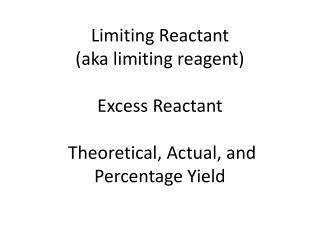DownloadDownload PresentationLimiting Reactant (aka limiting reagent)

# Limiting Reactant (aka limiting reagent)

Télécharger la présentation## Limiting Reactant (aka limiting reagent)

- - - - - - - - - - - - - - - - - - - - - - - - - - - E N D - - - - - - - - - - - - - - - - - - - - - - - - - - -
##### Presentation Transcript

1. Limiting Reactant(aka limiting reagent)Excess Reactant Theoretical, Actual, and Percentage Yield

2. Limiting Reactant(aka limiting reagent) Limiting reactant: The substance you run out of first, that is consumed first--The reactant that limits the amount of other reactant(s) that can combine and the amount of products that can form

3. Excess Reactant EXCESS REACTANT: A substance that is not used up completely in a reaction. It’s what is left over after you run out of the limiting reactant. It is present in excess, more than enough.

4. Theoretical, Actual, andPercentage Yield Theoretical yield: the calculated maximum amount of product from a given amount of reactant. In theory, the reaction should yield this amount. Actual yield: the measured amount of product actually obtained from a reaction Percentage yield: the ratio of actual yield to theoretical yield x 100% (like any percent)

5. S’mores: 20 chocolate chips 30 marshmallows 40 cookies You can make 10 s’mores based on the chocolate chips You can make 15 s’mores based on the marshmallows. You can make 40 s’mores based on the cookies. How many s’ mores can you make? 10 YIELD What do you run out first? Chocolate chips LIMITING REACTANT How much of the other substance are left over? If you make 10 s’mores, you use 20 marshmalllows and have 10 left. You use 10 cookies and have 30 left. EXCESS REACTANT

6. S’mores: How many s’ mores can you make? 10 YIELD What do you run out first? Chocolate chips LIMITING REACTANT How much of the other substance are left over? If you make 10 s’mores, you use 20 marshmalllows and have 10 left. You use 10 cookies and have 30 left. EXCESS REACTANT If you get sneaky and eat a chocolate chip and can only make 9 s’mores, you have not made the EXPECTED or THEORETICAL YIELD. TheACTUAL YIELD is 9/10 x 100% or 90%

7. Limiting reactant • Calculate in several ways • Choose a product and calculate the quantity of that substance that would be produced by each of the starting quantities of reactants. • Depending on the problem, you may calculate for moles (the easiest and shortest calculation), grams, particles, or volume. • The starting substance that yields the lowest quantity is the limiting reactant. • EXAMPLE

8. Limiting reactant • If no theoretical yield is required, you may use this shortcut. You are never required to use it. • Convert starting unit to moles • Use the coefficient for each starting substance to determine how many “reactions” you could get from each substance. • The starting substance that yields the fewest “reactions” is the limiting reactant. • This is similar to figuring out how many batches of a recipe you can make from the ingredients on hand. • EXAMPLE

9. Excess Reactant • Set up a stoichiometric calculation from your product (yield) or limiting reactant to the excess reactant to figure out how much of the excess reactant is actually consumed in the reaction. Calculate for the units asked for in the problem. • Subtract the amount of the excess substance actually consumed from the amount of the substance added. This is the excess reactant quantity. • Amount added-amount consumed = excess • Choose units based on your problem (moles, gram, particles, volume) EXAMPLE

10. Theoretical yield, actual yield, and percentage yield • Real world reactions do not always go to completion to produce the expected or theoretical yield. • The actual yield is given to you in the problem or determined experimentally. • Actual yield/ theoretical (expected) yield x 100% = Percentage yield • EXAMPLE